# 细分曲面奇异点处的光滑过渡Smooth Connection near Singular Points on Subdivision Surfaces

DOI: 10.12677/AAM.2017.69141, PDF, HTML, XML, 下载: 1,063  浏览: 1,648  国家自然科学基金支持

Abstract: This paper discusses G2 smooth connection near singular points on subdivision surfaces. A shape adjustable Catmull-Clark subdivision algorithm is present by introducing subdivision shape ad-justment parameter c(0≤c≤1). Based on this, aimed at the singular points in subdivision surface, based on the 2-ring of the singular points as control mesh, adopting the method of cyclic mapping, this paper proposes a G2 surface modeling method which is shape-adjustable. Then we can get the explicit solution of the Bezier control points. Compared with existing methods, the generated surface not only achieves the G2 continuous at singular points, but solves the problem of the curved surface design to be adjustable. The algorithm process and data structure are present. Corresponding examples are also given in this paper.

1. 引言

2. 一种形状可调的C-C细分曲面

(1) 几何点的产生

${f}_{i}^{\left(k+1\right)}=\frac{1}{4}\left({v}^{\left(k\right)}+{f}_{i}^{\left(k\right)}+{e}_{i}^{\left(k\right)}+{e}_{i+1}^{\left(k\right)}\right)$

${e}_{i}^{\left(k+1\right)}=\frac{{v}^{\left(k\right)}+{e}_{i}^{\left(k\right)}}{2}c+\frac{{f}_{i-1}^{\left(k+1\right)}+{f}_{i+1}^{\left(k+1\right)}}{2}\left(1-c\right)$

${v}^{\left(k+1\right)}=\frac{4cQ}{n}+\frac{8c\left(1-c\right)R}{n}+\frac{n-4c\left(2-c\right){v}^{\left(k\right)}}{n}$

(2) 拓扑结构的建立

$C=\left[\begin{array}{cccc}\frac{c}{2\left(1+c\right)}& \frac{1}{1+c}& \frac{c}{2\left(1+c\right)}& 0\\ -\frac{c\left(2-c\right)}{1+c}& 0& \frac{c\left(2-c\right)}{1+c}& 0\\ \frac{c\left(5-4c\right)}{2\left(1+c\right)}& \frac{\left(-2c+3\right)\left(c-2\right)}{2\left(1+c\right)}& \frac{4{c}^{2}-11c+6}{2\left(1+c\right)}& \frac{c\left(2c-1\right)}{2\left(1+c\right)}\\ \frac{c\left(c-1\right)}{1+c}& \frac{\left(c-1\right)\left(c-2\right)}{1+c}& -\frac{\left(c-1\right)\left(c-2\right)}{1+c}& \frac{c\left(1-c\right)}{1+c}\end{array}\right]$

3. 细分曲面奇异点处的G2连续性

3.1. 二阶几何连续条件

$\frac{{\partial }^{i+j}}{\partial {u}^{i}\partial {v}^{j}}P\left(t\right)=\frac{{\partial }^{i+j}}{\partial {s}^{i}\partial {r}^{j}}\stackrel{¯}{Q}\left(t\right),\text{\hspace{0.17em}}\text{\hspace{0.17em}}i+j=0,1,\cdots ,k.$

$\frac{{\partial }^{2}}{\partial {u}_{}^{2}}{P}_{i}^{}={p}_{2}\left(v\right)\frac{\partial }{\partial u}{P}_{i+1}+{q}_{2}\left(v\right)\frac{\partial }{\partial v}{P}_{i+1}+{p}_{1}^{2}\left(v\right)\frac{{\partial }^{2}}{\partial {u}^{2}}{P}_{i+1}+2{p}_{1}\left(v\right){q}_{1}\left(v\right)\frac{{\partial }^{2}}{\partial u\partial v}{P}_{i+1}+{q}_{1}^{2}\left(v\right)\frac{{\partial }^{2}}{\partial {v}^{2}}{P}_{i+1}.$

3.2. 初始控制网格的“混合细分”

$P\left(u,v\right)=UCH{C}^{\text{T}}{V}^{\text{T}}$

$A=CH{C}^{\text{T}}$$H$ 为双三次系数矩阵，则第 $k$ 个曲面片对应的系数矩阵为 ${A}_{k}={C}_{k}{H}_{k}{C}_{k}^{\text{T}}$ ，相应的参数调节矩阵为

${C}_{k}=\left[\begin{array}{cccc}\frac{{c}_{k}}{2\left(1+{c}_{k}\right)}& \frac{1}{1+{c}_{k}}& \frac{{c}_{k}}{2\left(1+{c}_{k}\right)}& 0\\ -\frac{{c}_{k}\left(2-{c}_{k}\right)}{1+{c}_{k}}& 0& \frac{{c}_{k}\left(2-{c}_{k}\right)}{1+{c}_{k}}& 0\\ \frac{{c}_{k}\left(5-4{c}_{k}\right)}{2\left(1+{c}_{k}\right)}& \frac{\left(-2{c}_{k}+3\right)\left({c}_{k}-2\right)}{2\left(1+{c}_{k}\right)}& \frac{4{c}_{k}^{2}-11{c}_{k}+6}{2\left(1+{c}_{k}\right)}& \frac{{c}_{k}\left(2{c}_{k}-1\right)}{2\left(1+{c}_{k}\right)}\\ \frac{{c}_{k}\left({c}_{k}-1\right)}{1+{c}_{k}}& \frac{\left({c}_{k}-1\right)\left({c}_{k}-2\right)}{1+{c}_{k}}& -\frac{\left({c}_{k}-1\right)\left({c}_{k}-2\right)}{1+{c}_{k}}& \frac{{c}_{k}\left(1-{c}_{k}\right)}{1+{c}_{k}}\end{array}\right]$

${T}_{i}=\left[\begin{array}{cccc}\frac{\partial {P}_{i}}{\partial u}& \frac{\partial {P}_{i}}{\partial v}& \frac{{\partial }^{2}{P}_{i}}{\partial {u}^{2}}& \begin{array}{cccc}\frac{{\partial }^{2}{P}_{i}}{\partial u\partial v}& \frac{{\partial }^{2}{P}_{i}}{\partial {v}^{2}}& \frac{{\partial }^{3}{P}_{i}}{\partial {u}^{2}\partial v}& \begin{array}{cc}\frac{{\partial }^{3}{P}_{i}}{\partial u\partial {v}^{2}}& \frac{{\partial }^{4}{P}_{i}}{\partial {u}^{2}\partial {v}^{2}}\end{array}\end{array}\end{array}\right]$

${T}_{i+1}=\left[\begin{array}{cccc}\frac{\partial {P}_{i+1}}{\partial u}& \frac{\partial {P}_{i+1}}{\partial v}& \frac{{\partial }^{2}{P}_{i+1}}{\partial {u}^{2}}& \begin{array}{cccc}\frac{{\partial }^{2}{P}_{i+1}}{\partial u\partial v}& \frac{{\partial }^{2}{P}_{i+1}}{\partial {v}^{2}}& \frac{{\partial }^{3}{P}_{i+1}}{\partial {u}^{2}\partial v}& \begin{array}{cc}\frac{{\partial }^{3}{P}_{i+1}}{\partial u\partial {v}^{2}}& \frac{{\partial }^{4}{P}_{i+1}}{\partial {u}^{2}\partial {v}^{2}}\end{array}\end{array}\end{array}\right]$

${\Theta }_{i}$ 为曲面片 ${P}_{i}$${P}_{i+1}$ 之间的参数化矩阵，则由二阶几何连续性定义可以得到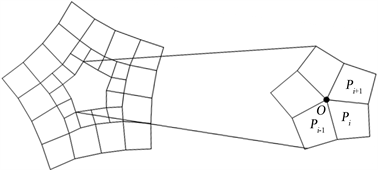Figure 1. N-sided hole formed by mingle subdivision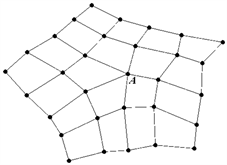Figure 2. 2-ring around singular vertex on mesh

${T}_{i}={T}_{i+1}\cdot {\Theta }_{i}$

${T}_{0}={T}_{0}\cdot {\Theta }_{n-1}\cdot {\Theta }_{n-2}\cdots {\Theta }_{1}\cdot {\Theta }_{0}$

$I={\Theta }_{n-1}\cdot {\Theta }_{n-2}\cdots {\Theta }_{1}\cdot {\Theta }_{0}$

3.2.1.内部边界的连续性

$\left\{\begin{array}{l}x=\left(\mathrm{cos}\frac{\text{2π}}{n}\right)\left[\left(1-u\right)v+\mathrm{sec}\frac{\text{2π}}{n}\right]\\ y=\left(\mathrm{sin}\frac{\text{2π}}{n}\right)\left[\left(1-u\right)v+\left(\mathrm{sec}\frac{\text{2π}}{n}\right)uv\right]\end{array}$

${r}_{n}$ 是以原点为中心 $\frac{\text{2π}}{n}$ 为单位的一个逆时针旋转变换

${r}_{n}\left(u,v\right)=\left[\begin{array}{cc}\mathrm{cos}\frac{\text{2π}}{n}& -\mathrm{sin}\frac{\text{2π}}{n}\\ \mathrm{sin}\frac{\text{2π}}{n}& \mathrm{cos}\frac{\text{2π}}{n}\end{array}\right]\left[\begin{array}{c}u\\ v\end{array}\right]$

$I={\left({\Phi }_{n}^{-1}\cdot {R}_{n}^{-1}\cdot {\Phi }_{n}\right)}^{n}={\Phi }_{n}^{-1}\cdot {\left({R}_{n}^{-1}\right)}^{n}\cdot {\Phi }_{n}$ (1)

${P}_{i}\left(0,t\right)-{P}_{i+1}\left(t,0\right)=0$ (2)

${c}_{n}\left(1-t\right){P}_{i+1}^{u}\left(t,0\right)-{P}_{i+1}^{v}\left(t,0\right)-{c}_{n}\left(t-1\right){P}_{i}{}^{v}\left(0,t\right)-{P}_{i}^{u}\left(0,t\right)=0$ (3) $\begin{array}{l}2{c}_{n}^{2}\left(1-t\right)\left({P}_{i+1}^{v}\left(t,0\right)-{P}_{i}^{u}\left(0,t\right)\right)+2{c}_{n}\left(1-t\right)\left(1+{c}_{n}\left(1-t\right)\right)\left({P}_{i+1}^{uv}\left(t,0\right)-{P}_{i}^{uv}\left(0,t\right)\right)\\ -\left(1+{c}_{n}\left(1-t\right)\right)\left({P}_{i+1}^{vv}\left(t,0\right)-{P}_{i}^{uu}\left(0,t\right)\right)=0\end{array}$ (4)

${p}_{00}^{i}={p}_{07}^{i+1},{p}_{01}^{i}={p}_{17}^{i+1},{p}_{02}^{i}={p}_{27}^{i+1},{p}_{03}^{i}={p}_{37}^{i+1},$

${p}_{04}^{i}={p}_{47}^{i+1},{p}_{05}^{i}={p}_{57}^{i+1},{p}_{06}^{i}={p}_{77}^{i+1},{p}_{07}^{i}={p}_{77}^{i+1}.$

${c}_{n}\left(-{\alpha }_{1}-2{\alpha }_{2}+3{\alpha }_{3}-7{\alpha }_{4}+5{\alpha }_{5}-21{\alpha }_{6}+7{\alpha }_{7}-{\alpha }_{8}\right)$ $+\left({\beta }_{1}-{\beta }_{2}+{\beta }_{3}-{\beta }_{4}+{\beta }_{5}-{\beta }_{6}+{\beta }_{7}-{\beta }_{8}\right)=0$ .

${c}_{n}\left(7{\alpha }_{1}\text{+}13{\alpha }_{2}-17{\alpha }_{3}+31{\alpha }_{4}-19{\alpha }_{5}+57{\alpha }_{6}-13{\alpha }_{7}+{\alpha }_{8}\right)$ $+\left(-7{\beta }_{1}+6{\beta }_{2}-5{\beta }_{3}+4{\beta }_{4}-3{\beta }_{5}+2{\beta }_{6}-{\beta }_{7}\right)=0.$ ${c}_{n}\left(-21{\alpha }_{1}-36{\alpha }_{2}+40{\alpha }_{3}-54{\alpha }_{4}+27{\alpha }_{5}-51{\alpha }_{6}+6{\alpha }_{7}\right)$ $+\left(21{\beta }_{1}-15{\beta }_{2}+10{\beta }_{3}-6{\beta }_{4}+3{\beta }_{5}-{\beta }_{6}\right)=0.$ ${c}_{n}\left(35{\alpha }_{1}+55{\alpha }_{2}-50{\alpha }_{3}+46{\alpha }_{4}-17{\alpha }_{5}+15{\alpha }_{6}+6{\alpha }_{7}\right)+\left(-35{\beta }_{1}+20{\beta }_{2}-10{\beta }_{3}+4{\beta }_{4}-{\beta }_{5}\right)=0.$ ${c}_{n}\left(-35{\alpha }_{1}-50{\alpha }_{2}+35{\alpha }_{3}-19{\alpha }_{4}+4{\alpha }_{5}\right)+\left(35{\beta }_{1}-15{\beta }_{2}+5{\beta }_{3}-{\beta }_{4}\right)=0.$ ${c}_{n}\left(21{\alpha }_{1}+27{\alpha }_{2}-13{\alpha }_{3}+3{\alpha }_{4}\right)+\left(-21{\beta }_{1}+6{\beta }_{2}-{\beta }_{3}\right)=0.$

${c}_{n}\left(-7{\alpha }_{1}-8{\alpha }_{2}+2{\alpha }_{3}\right)+\left(7{\beta }_{1}-{\beta }_{2}\right)=0.$

${c}_{n}\left({\alpha }_{1}+{\alpha }_{2}\right)-{\beta }_{1}=0.$

${\alpha }_{1}=-7{p}_{00}^{i+1}-7{p}_{00}^{i},\text{\hspace{0.17em}}\text{\hspace{0.17em}}{\alpha }_{2}=7{p}_{10}^{i+1}+7{p}_{01}^{i},\text{\hspace{0.17em}}\text{\hspace{0.17em}}{\alpha }_{3}=21{p}_{20}^{i+1}+21{p}_{02}^{i},\text{\hspace{0.17em}}\text{\hspace{0.17em}}{\alpha }_{4}=35{p}_{30}^{i+1}+35{p}_{03}^{i},$

${\alpha }_{5}=35{p}_{40}^{i+1}+35{p}_{04}^{i},\text{\hspace{0.17em}}\text{\hspace{0.17em}}{\alpha }_{6}=7{p}_{50}^{i+1}+7{p}_{05}^{i},\text{\hspace{0.17em}}\text{\hspace{0.17em}}{\alpha }_{7}=7{p}_{60}^{i+1}+7{p}_{06}^{i},\text{\hspace{0.17em}}\text{\hspace{0.17em}}{\alpha }_{8}=7{p}_{70}^{i+1}+7{p}_{07}^{i}.$

${\beta }_{1}=7\left({p}_{00}^{i+1}-{p}_{01}^{i+1}\right)+7\left({p}_{10}^{i}-{p}_{00}^{i}\right),\text{\hspace{0.17em}}\text{\hspace{0.17em}}{\beta }_{2}=49\left({p}_{10}^{i+1}-{p}_{11}^{i+1}\right)+49\left({p}_{11}^{i}-{p}_{01}^{i}\right),$

${\beta }_{3}=147\left({p}_{20}^{i+1}-{p}_{21}^{i+1}\right)+147\left({p}_{12}^{i}-{p}_{02}^{i}\right),\text{\hspace{0.17em}}\text{\hspace{0.17em}}{\beta }_{4}=245\left({p}_{30}^{i+1}-{p}_{31}^{i+1}\right)+245\left({p}_{13}^{i}-{p}_{03}^{i}\right),$

${\beta }_{5}=245\left({p}_{40}^{i+1}-{p}_{41}^{i+1}\right)+245\left({p}_{14}^{i}-{p}_{04}^{i}\right),\text{\hspace{0.17em}}\text{\hspace{0.17em}}{\beta }_{6}=147\left({p}_{50}^{i+1}-{p}_{51}^{i+1}\right)+147\left({p}_{15}^{i}-{p}_{05}^{i}\right),$

${\beta }_{7}=7\left({p}_{60}^{i+1}-{p}_{61}^{i+1}\right)+7\left({p}_{16}^{i}-{p}_{06}^{i}\right),\text{\hspace{0.17em}}\text{\hspace{0.17em}}{\beta }_{8}=7\left({p}_{70}^{i+1}-{p}_{71}^{i+1}\right)+7\left({p}_{17}^{i}-{p}_{07}^{i}\right).$

3.2.2. 外部边界的连续性

$q\left(u,v\right)=\left[\begin{array}{cc}1& 0\\ 0& -1\end{array}\right]\left[\begin{array}{c}u\\ v\end{array}\right]$$s\left(u,v\right)=\left[\begin{array}{cc}0& 1\\ 1& 0\end{array}\right]\left[\begin{array}{c}u\\ v\end{array}\right]$

$Q={\left[\begin{array}{ccccc}1& 0& & & \\ 0& -1& & & \\ & & \ddots & & \\ & & & 1& 0\\ & & & 0& -1\end{array}\right]}_{n×n}$$S={\left[\begin{array}{ccccc}0& 1& & & \\ 1& 0& & & \\ & & \ddots & & \\ & & & 0& 1\\ & & & 1& 0\end{array}\right]}_{n×n}$

$I=\left(Q\cdot {\psi }_{n}\cdot S\cdot {\left({R}_{n}^{-1}\cdot {\phi }_{n}\right)}^{-1}\cdot {\phi }_{n}\cdot {\psi }_{n}^{-1}\right)\cdot \left(Q\cdot {\psi }_{m}\cdot S\cdot {\left({R}_{m}^{-1}\cdot {\phi }_{m}\right)}^{-1}\cdot {\phi }_{m}\cdot {\psi }_{m}^{-1}\right)$

${\psi }_{n}\left(u,v\right)={\psi }_{n}\left(v,u\right)$ ，则 $S\cdot {\psi }_{n}\cdot S={\psi }_{n}$ ，对于第二类点有

$I={\psi }_{k}\cdot {\left(S\cdot {\psi }_{k}\cdot S\right)}^{-1}\cdot {\psi }_{l}\cdot {\left(S\cdot {\psi }_{l}\cdot S\right)}^{-1}\cdot {\psi }_{m}\cdot {\left(S\cdot {\psi }_{k}\cdot S\right)}^{-1}\cdot {\psi }_{n}\cdot {\left(S\cdot {\psi }_{n}\cdot S\right)}^{-1}$

$y\left(u,v\right)={b}^{4}{\left(v\right)}^{\text{T}}\left[\begin{array}{cccc}0& 0& 0& 0\\ \frac{1}{4}& \frac{5{c}_{n}^{3}-3{c}_{n}^{2}-15{c}_{n}+18}{12\left({c}_{n}-2\right)\left(2{c}_{n}-3\right)}& \frac{{c}_{n}^{2}+2{c}_{n}-6}{12\left({c}_{n}-2\right)}& \frac{1}{4}\\ \frac{1}{2}& \frac{{c}_{n}+3}{6}& \frac{{c}_{n}^{2}+3{c}_{n}-9}{9\left({c}_{n}-2\right)}& \frac{1}{2}\\ \frac{3}{4}& \frac{{c}_{n}+9}{12}& \frac{{c}_{n}+9}{12}& \frac{3}{4}\\ 1& 1& 1& 1\end{array}\right]{b}^{3}\left(u\right)$

${b}^{k}=\frac{1}{36}{Q}^{\text{T}}AQ$

$A$ 为“混合细分”矩阵。

${A}_{k}=\left[\begin{array}{cccc}1& {a}_{10}^{k+2}& {a}_{11}^{k+1}& {a}_{12}^{k+1}\\ {a}_{10}^{k-1}& {a}_{00}^{k}& {a}_{10}^{k+1}& {a}_{20}^{k+1}\\ {a}_{11}^{k-1}& {a}_{10}^{k}& {a}_{11}^{k}& {a}_{12}^{k}\\ {a}_{12}^{k-1}& {a}_{20}^{k}& {a}_{20}^{k}& {a}_{22}^{k}\end{array}\right]$

${B}_{i}\left(u,v\right)=\frac{1}{36}{b}^{3}\left(u\right){Q}^{\text{T}}\left[\begin{array}{cccc}1& {a}_{10}^{k+2}& {a}_{11}^{k+1}& {a}_{12}^{k+1}\\ {a}_{10}^{k-1}& {a}_{00}^{k}& {a}_{10}^{k+1}& {a}_{20}^{k+1}\\ {a}_{11}^{k-1}& {a}_{10}^{k}& {a}_{11}^{k}& {a}_{12}^{k}\\ {a}_{12}^{k-1}& {a}_{20}^{k}& {a}_{21}^{k}& {a}_{22}^{k}\end{array}\right]Q{\left({b}^{3}\left(v\right)\right)}^{\text{T}}$

$\frac{{\partial }^{j}}{\partial {u}^{j}}{P}_{i}\left(1,t\right)=\frac{{\partial }^{j}}{\partial {u}^{j}}\left({B}_{i}\circ {\psi }_{n}\right)\left(1,t\right),\text{\hspace{0.17em}}j=0,1,2.$

${P}_{i}\left(1,t\right)$${B}_{i}\left(1,t\right)$$\frac{\partial }{\partial u}{P}_{i}\left(1,t\right)$$\frac{\partial }{\partial x}{B}_{i}\left(1,t\right)$$\frac{\partial }{\partial y}{B}_{i}\left(1,t\right)$$\frac{{\partial }^{2}}{\partial {x}^{2}}{B}_{i}\left(1,t\right)$$\frac{{\partial }^{2}}{\partial xy}{B}_{i}\left(1,t\right)$$\frac{{\partial }^{2}}{\partial {y}^{2}}{B}_{i}\left(1,t\right)$ 。同时，由已知条件可以得到

$\frac{\partial }{\partial u}{\psi }_{n,x}\left(1,t\right)=-\frac{1}{2}{\left(1-t\right)}^{3}-\frac{3\left({c}_{n}+1\right)}{2}t{\left(1-t\right)}^{2}-\frac{3\left({c}_{n}+1\right)}{2}{t}^{2}\left(1-t\right)-\frac{1}{2}{t}^{3}$

$\frac{\partial }{\partial u}{\psi }_{n,y}=\frac{-{c}_{n}^{2}+{c}_{n}}{{c}_{n}-2}t{\left(1-t\right)}^{3}+\frac{-2{c}_{n}^{2}+\frac{33}{2}{c}_{n}-27}{{c}_{n}-2}{t}^{2}{\left(1-t\right)}^{2}-\frac{3\left({c}_{n}+9\right)}{2}{t}^{3}\left(1-t\right)$

$\frac{{\partial }^{2}}{\partial {u}^{2}}{\psi }_{n,x}\left(1,t\right)=-9{\left(1-t\right)}^{3}-3\left({c}_{n}+9\right)t{\left(1-t\right)}^{2}+\frac{3\left({c}_{n}^{2}+3{c}_{n}-6\right)}{{c}_{n}-2}{t}^{2}\left(1-t\right)-9{t}^{3}$

$\frac{{\partial }^{2}}{\partial {u}^{2}}{\psi }_{n,y}\left(1,t\right)=\frac{2{c}_{n}^{3}+2{c}_{n}^{2}}{\left({c}_{n}-2\right)\left(2{c}_{n}-3\right)}t{\left(1-t\right)}^{3}-\frac{2{c}_{n}^{2}}{{c}_{n}-2}{t}^{2}{\left(1-t\right)}^{2}-3{c}_{n}{t}^{3}\left(1-t\right)$

3.3. Bézier控制点的求解

$CP=WA$

$\left\{\begin{array}{l}\underset{i=0}{\overset{n-1}{\sum }}{c}_{i}^{\text{T}}{\lambda }_{i}+\underset{i=0}{\overset{n-1}{\sum }}E{p}_{i}=0\hfill \\ \underset{i=0}{\overset{n-1}{\sum }}{c}_{i}{p}_{i}=\underset{i=0}{\overset{n-1}{\sum }}{\omega }_{i}{a}_{i}\hfill \end{array}$

Euler系数 ${C}_{n}^{{k}_{l}}=\mathrm{cos}\frac{2\text{π}{k}_{l}}{n}$${S}_{n}^{{k}_{l}}=\mathrm{sin}\frac{2\text{π}{k}_{l}}{n}$ 则有

${\stackrel{^}{\rho }}_{j}=\frac{1}{n}\underset{k=0}{\overset{n-1}{\sum }}{\tau }_{k}{\rho }_{k}$ (5)

${p}_{i},{c}_{i},{\lambda }_{i},{\omega }_{i},{a}_{i}$ 代入(5)式即可得到 ${\stackrel{^}{p}}_{j},{\stackrel{^}{c}}_{j},{\stackrel{^}{\lambda }}_{j},{\stackrel{^}{\omega }}_{j},{\stackrel{^}{a}}_{j}$

4. 曲面造型结果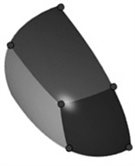(a)3价奇异点局部网格(b)3奇异点的控制顶点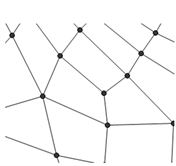(c)3价奇异点的拟合

Figure 3. 3-sided hole filling(a)5价奇异点局部网格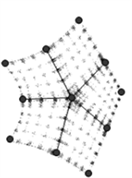(b)5奇异点的控制顶点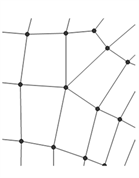(c)5价奇异点的拟合

Figure 4. 5-sided hole filling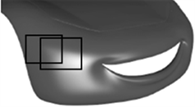(a) 进行“混合细分”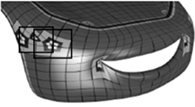(b) 对n边洞进行填充(c) G2连续曲面

Figure 5. Surface modeling with G2 continuity

5. 小结

  Cashman, T.J. (2010) NURBS-Compatible Subdivision Surfaces. University of Cambridge Compute Laboratory, 773-873.  Cashman, T.J., Dodgson, N.A. and Sabin, M.A. (2009) Selective Knot Insertion for Symmetric, Non-Uniform, Refine and Smooth B-Splines Subdivision. Computer Aided Geometric Design, 26, 472. https://doi.org/10.1016/j.cagd.2008.11.002  刘浩, 廖文和. 用C-C细分方法和流行方法构造G2连续的自由型曲面[J]. 计算机辅助设计与图形学学报, 2005, 17(4): 644-649.  Loop, C. (2004) Second Order Smoothness over Extraordinary Vertices. Proceedings of the 2004 Eurographics/ACM Siggraph Symposium on Geometry Processing, 71, 165- 174. https://doi.org/10.1145/1057432.1057454  王卫民, 罗国明, 张纪文, 等. 一种可调的Catmull-Clark细分曲面[J]. 工程图学学报, 2002, 23(3): 115-121.  王国谨, 汪国昭, 郑建民. 计算机辅助几何设计[M]. 北京: 高等教育出版社; 海德堡: 施普林格出版社, 2001.  邓群毅. 关于循环矩阵的推广及应用[D]: [博士学位论文]. 宁波: 宁波大学, 2012.  Eck, M. and Hoop, H. (1996) Automatic Reconstruction of B-Spline Surfaces of Arbitrary Topological Type. Computer Graphics, 30, 325-334. https://doi.org/10.1145/237170.237271  Yong, J.-H. and Cheng, F.H. (2005) Adaptive Subdivision of Cat-mull-Clark Subdivision Surfaces. Computer-Aided Design & Applications, 2, 253-261. https://doi.org/10.1080/16864360.2005.10738373  Li, W.-X., Zhang, D. and Xu, Z.-Q. (2002) Palmprint Recognition Based on Fourier Transform. Journal of Software, 13, 879-886.